# VC: Everything about Scatter Plots

Scatterplots are one of the most popular visualization techniques in the world. Its purposes are recognizing clusters and correlations in ‘pairs’ of variables. There are many variations of scatter plots. We will look at some of them.

Strip Plots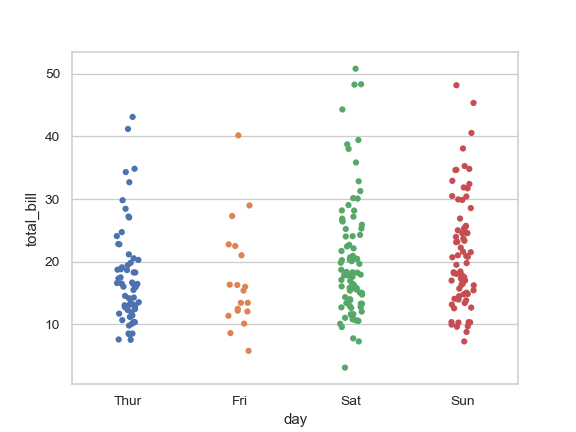Strip Plots, Seaborn Documentation

Scatter plots in which one attribute is categorical are called ‘strip plots’. Since it is hard to see the data points when we plot the data points as a single line, we need to slightly spread the data points, you can check the above and we can also divide the data points based on the given label.

Scatterplot Matrices (SPLOM)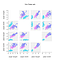Scatterplot Matrices

SPLOM produce scatterplots for all pairs of variables and place them into a matrix. Total unique scatterplots are (p²-p)/2. The diagonal is filled with KDE or histogram most of the time. As you can see, there is an order of scatterplots. Does the order matter? It cannot affect the value of course but it can affect the perception of people.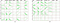Ordering is matter, Image taken from [Peng et al. 2004]

Therefore we need to consider the order of it. Peng suggests the ordering that similar scatterplots are located close to each other in his work in 2004 [Peng et al. 2004]. They distinguish between high-cardinality and low cardinality(number of possible values > number of points means high cardinality.) and sort low-cardinality by a number of values. They rate the ordering of high-cardinality dimensions based on their correlation. Pearson Correlation Coefficient is used for sorting.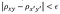clutter measure

We find all other pairs of x,y scatter plots with clutter measure. It calculates all correlation and compares it with each pair (x,y ) of high-cardinality dimensions. If its results are smaller than the threshold we choose that scatter plot as an important one. However, it takes a lot of computing power because its big-o-notation is O(p² * p!). They suggest random swapping, it chooses the smallest one and keeps it and again and again.

Selecting Good Views

Correlation is not enough to choose the nice scatterplots when we are trying to find out the cluster based on the given label or we can get the label from clustering.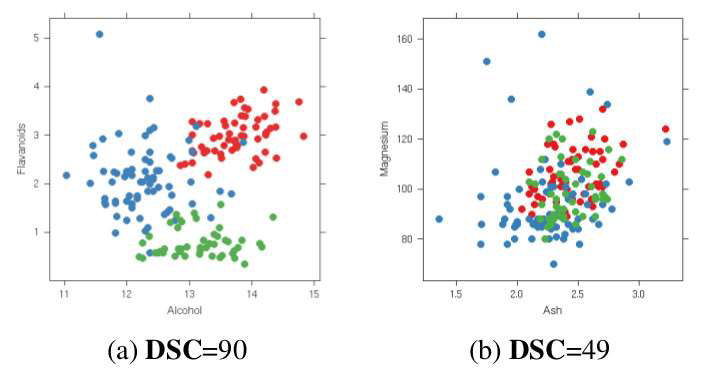Histogram and DSC, [Sips et al. 2009]

If you don’t have given labels in the left graph, you can pick x-axis projection or y-axis projection because there are no many differences but there are labels. Therefore, we can know the x-axis projection is correct., DSC is introduced with respect to this view that the method checks how good our scatterplot is. More good separation, more good scatterplots.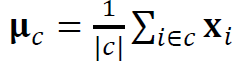Cluster center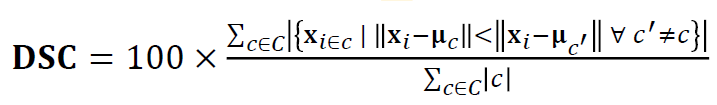The equation to calculate DSC

First of all, we calculate the center of each cluster and measure the distance between each data point and each cluster center. If the distance from its own cluster is shorter than other clusters distance, we increase the cardinality and we normalized it by the number of clusters and multiply 100. This method is similar to the k-means clustering method. Since it only considers distance, it has a limitation to applying.

Distribution Consistency (DC)

DC is the upgrade(?) version of DSC. DC measures the score based on penalizing local entropy in high-density regions. DSC assumes the particular cluster shapes but DC does not assume the shapes.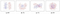Example of DC [Sips et al. 2009]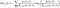Entropy

This equation is from information theory and it considers how much information in a specific distribution. The data should be estimated using KDE before we apply the entropy function, p(x,y) means the KDE. This equation means it gives smaller(Look at the minus) when the region we measure is mixed with other clusters and its minimum is 0 and the maximum is log2|C|.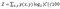Normalization function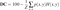DC score function

We calculated the entropy with KDE and we don’t want to calculate the whole region at the same weight because there are many vacant regions. Finally, we normalize the results. This gives the DC score. We can choose scatterplots based on thresholds that we can choose.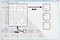WHO example of HIV risk groups

This dataset is from the WHO, 194 countries, 159 attributes, and 6 HIV risk groups. They focus on DC > 80 and they can eliminate 97% of the plots. It is a highly efficient method.

Other than these methods that it only considers the clusters, there are many ways to consider other specific patterns, e.g. fraction of outliers, sparsity, convexity, and e.t.c. You can take a look at [Wilkinson et al. 2006]. PCA also can be used as an alternative way to group similar plots together.

SPLOM Navigation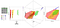The 3D transition between neighboring views. [Elmqvist et al. 2008]

Since the SPLOM shares one axis with the neighboring plots, it is possible to project on to 3D space.

The limitation of scatterplots: Overdraw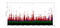Overdraw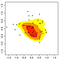KDE solution

Too many data points lead to overdraw. We can solve this with KDE but it becomes no longer see individual points. The second problem is high dimensional data because it gives too many scatterplots. We discussed the solution of the second problem. Now we are going to look at the first problem.

Splatterplots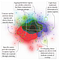Splatterplots [Mayorga/Gleicher 2013]

Splatterplots properly combine the KDE and Scatterplots. The high-density region is represented by colors and the low-density region is represented by a single data point. We need to choose a proper kernel width for KDE. Splatterplots define the kernel width in screen space, how many data points in the unit screen space. However, we need to choose the threshold by ourselves.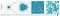Zoom of splatter plots [Mayorga / Gleicher 2013]

If clusters are mixed, then colors are matter. High luminance and saturation can cause the miss perception that people can recognize the mixed cluster as a different cluster. Therefore, we need to reduce the saturation and luminance to indicate it is mixed clusters.

This post is published on 9/2/2020.

Jeheon Park, Student, B-it (RWTH Aachen & Bonn University Information Technology Center), Germany, South Korean, Looking for Master Thesis Internship.

## More from Jeheonpark

Jeheon Park, Student, B-it (RWTH Aachen & Bonn University Information Technology Center), Germany, South Korean, Looking for Master Thesis Internship.